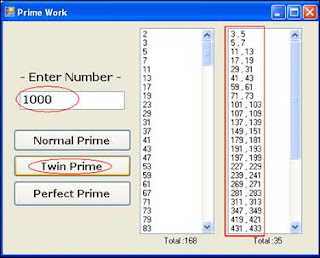# Playing with Numbers — 1

Finding Perfect Numbers and Twin Prime Numbers Programmatically

Description:

n Prime Numbers:

A Prime Number can be divided evenly only by 1 or itself. And it must be a whole number greater than 1.

Example: 2, 3, 5, 7, 11, etc.

n Twin Prime Numbers:

A pair of prime numbers that differ by 2 (successive odd numbers that are both Prime numbers).

Examples: (3, 5), (5, 7), (11, 13),…

It is not known whether the set of twin prime numbers ends or not.

n Perfect Prime Numbers :

Any positive integer that is equal to the sum of its distinct proper factors (factors other than the number itself).

Example: 6 (proper factors: 1, 2, 3) is a Perfect number because 1+2+3=6.

Example: 28 (proper factors: 1, 2, 4, 7, 14) is also a Perfect number, because 1+2+4+7+14=28.

Justification:

n To calculate Twin primes we need to compare the two successive prime numbers, if the result is 2 or -2 (depends upon the condition taken) then those two numbers can said to be the Twin Prime numbers. (See Figure 1)Figure 1

n To calculate Perfect prime numbers we need to compare the sum of the distinct proper factors of the any positive integer to itself, if both are equal that digit is the Perfect Number. (See Figure 2)Figure 2

Regards,
Mayur Dighe
http://ImmortalDotNet.blogspot.com
http://MayurDighe.wordpress.com